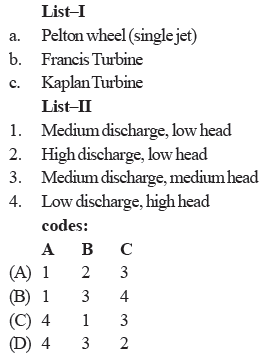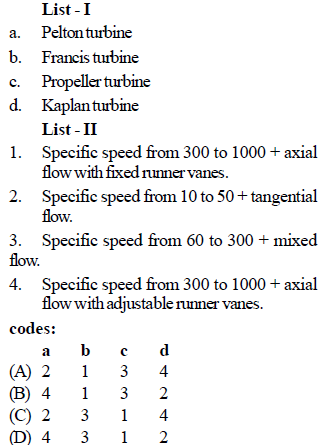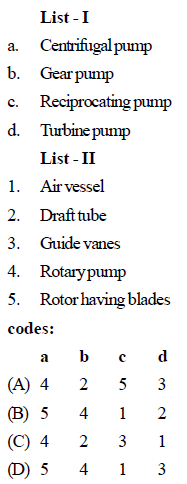Courses

# Test: Fluid Mechanics Level - 5

## 25 Questions MCQ Test Civil Engineering SSC JE (Technical) | Test: Fluid Mechanics Level - 5

Description
This mock test of Test: Fluid Mechanics Level - 5 for Civil Engineering (CE) helps you for every Civil Engineering (CE) entrance exam. This contains 25 Multiple Choice Questions for Civil Engineering (CE) Test: Fluid Mechanics Level - 5 (mcq) to study with solutions a complete question bank. The solved questions answers in this Test: Fluid Mechanics Level - 5 quiz give you a good mix of easy questions and tough questions. Civil Engineering (CE) students definitely take this Test: Fluid Mechanics Level - 5 exercise for a better result in the exam. You can find other Test: Fluid Mechanics Level - 5 extra questions, long questions & short questions for Civil Engineering (CE) on EduRev as well by searching above.
QUESTION: 1

Solution:
QUESTION: 2

Solution:
QUESTION: 3

### Match List–I with List–II and select the correct answer using the codes given below the lists:Solution:
QUESTION: 4

As water flows through the runner of a reaction turbine, pressure acting on it would vary from

Solution:
QUESTION: 5

Which one of the following turbines is used in underwater power stations ?

Solution:
QUESTION: 6

The correct sequence of the given hydraulic turbines in decreasing order of their specific speeds is

Solution:
QUESTION: 7

The force of impingement of a jet on a vane increases if the

Solution:
QUESTION: 8

Which one of the following graphs correctly represents the relations between Head and Specific speed for kaplan and Francis turbine ?

Solution:
QUESTION: 9

What should be the ratio of blade speed to jet speed for the maximum efficiency of a Pelton wheel ?

Solution:
QUESTION: 10

Which of the following water turbine does not require a draft tube ?

Solution:
QUESTION: 11

What does Euler's equation of turbo machines relate to ?

Solution:
QUESTION: 12

Match List–I with List – II and select the correct answer using the codes given below the lists:Solution:
QUESTION: 13

A jet of oil with relative density 0.7 strikes normally a plate with a velocity of 10 m/s. The jet has an area of 0.03 m2. The force exerted on the plate by the jet is

Solution:
QUESTION: 14

A Kaplan turbine is a

Solution:
QUESTION: 15

Hydraulic ram is pump which worked on the principle of

Solution:

The hydraulic ram is a pump which raises water without any external power for its operation. One essential requirement for the satisfactory operation ofa hydraulic ram is the availability of a large quantity of water with a small positive head or height. This large quantity of water at a small height is sufficient to lift small quantity of water to a greater height. It works on the principle of Water Hammer.

QUESTION: 16

Match List -I (Type of pumps) with List–II (Associated features) and select the correct answer using the codes given below the lists:Solution:
QUESTION: 17

Priming in necessary in

Solution:
QUESTION: 18

The vanes of a centrifugal pump are generally

Solution:
QUESTION: 19

Why is an air vessel used in a reciprocating pump?

Solution:
QUESTION: 20

Why is multi-staging in centrifugal pumps used?

Solution:
QUESTION: 21

For a given centrifugal pump

Solution:
QUESTION: 22

A Pelton wheel is

Solution:
QUESTION: 23

An impulse turbine is used for

Solution:
QUESTION: 24

The jet ratio is defined as the ratio of the

Solution:
QUESTION: 25

The function of guide vanes in a reaction turbine is to

Solution: